Скачать презентацию Algorithms Winter 2012 The study of algorithms is

2710a199086938ebcc19af167282e914.ppt

• Количество слайдов: 24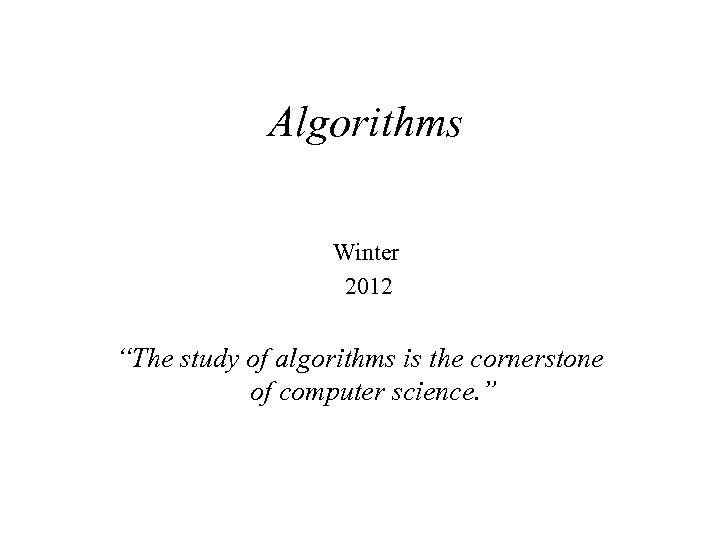Algorithms Winter 2012 “The study of algorithms is the cornerstone of computer science. ”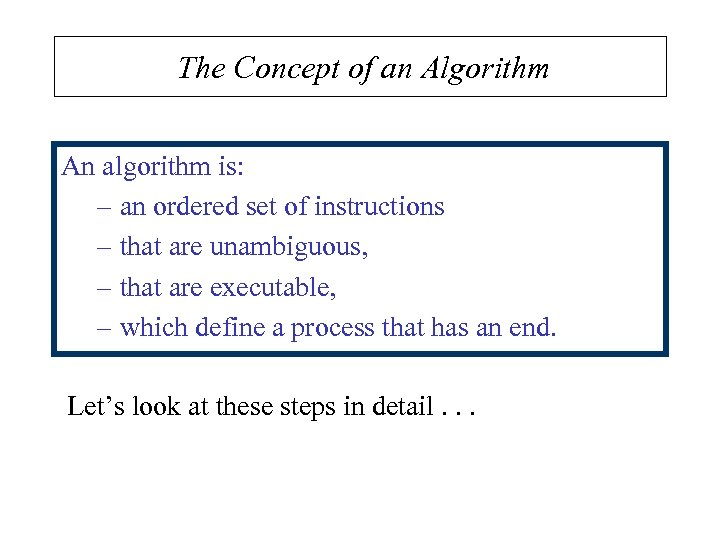The Concept of an Algorithm An algorithm is: – an ordered set of instructions – that are unambiguous, – that are executable, – which define a process that has an end. Let’s look at these steps in detail. . .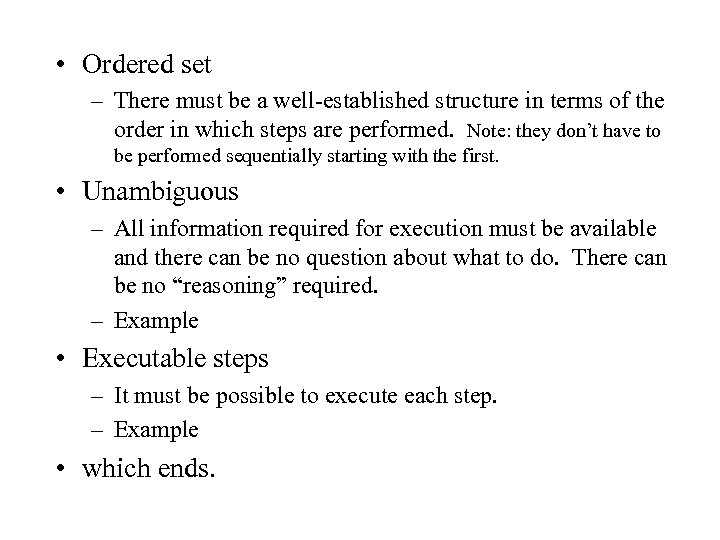• Ordered set – There must be a well-established structure in terms of the order in which steps are performed. Note: they don’t have to be performed sequentially starting with the first. • Unambiguous – All information required for execution must be available and there can be no question about what to do. There can be no “reasoning” required. – Example • Executable steps – It must be possible to execute each step. – Example • which ends.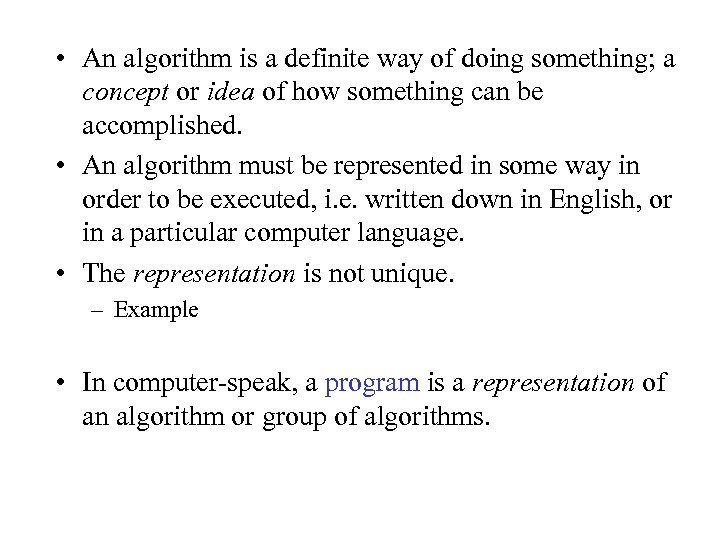• An algorithm is a definite way of doing something; a concept or idea of how something can be accomplished. • An algorithm must be represented in some way in order to be executed, i. e. written down in English, or in a particular computer language. • The representation is not unique. – Example • In computer-speak, a program is a representation of an algorithm or group of algorithms.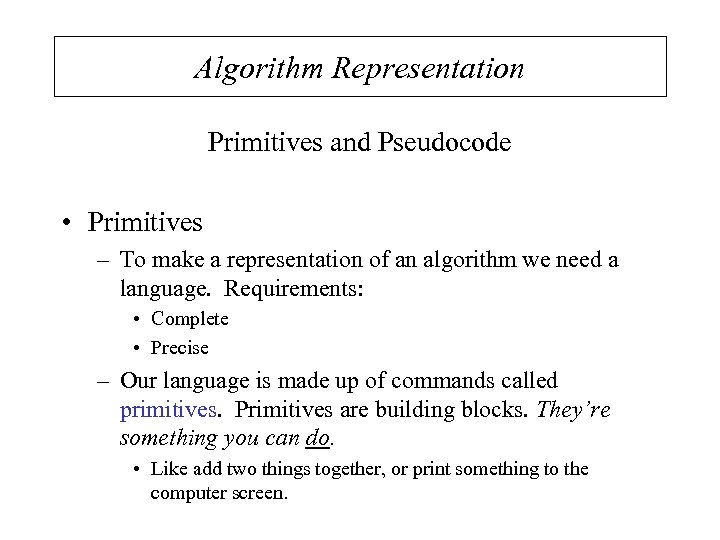Algorithm Representation Primitives and Pseudocode • Primitives – To make a representation of an algorithm we need a language. Requirements: • Complete • Precise – Our language is made up of commands called primitives. Primitives are building blocks. They’re something you can do. • Like add two things together, or print something to the computer screen.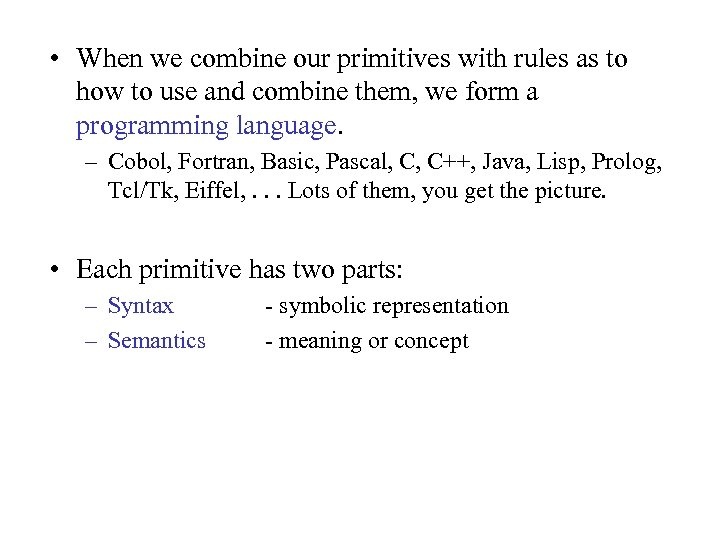• When we combine our primitives with rules as to how to use and combine them, we form a programming language. – Cobol, Fortran, Basic, Pascal, C, C++, Java, Lisp, Prolog, Tcl/Tk, Eiffel, . . . Lots of them, you get the picture. • Each primitive has two parts: – Syntax – Semantics - symbolic representation - meaning or concept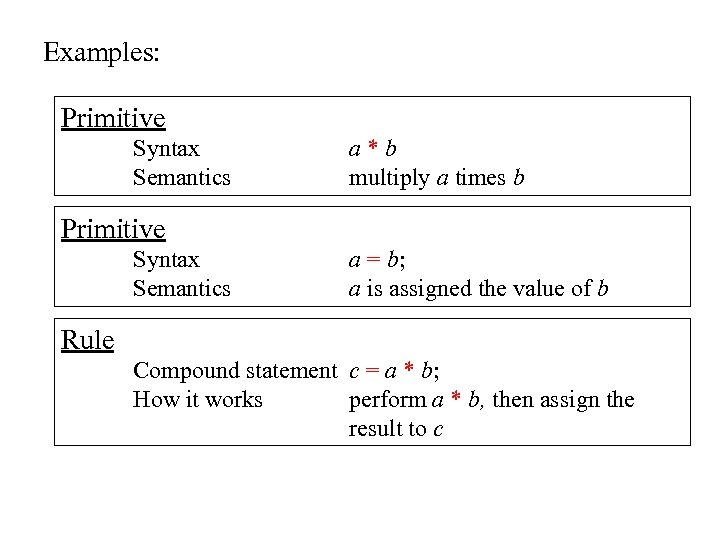Examples: Primitive Syntax Semantics a*b multiply a times b Primitive Syntax Semantics a = b; a is assigned the value of b Rule Compound statement c = a * b; How it works perform a * b, then assign the result to c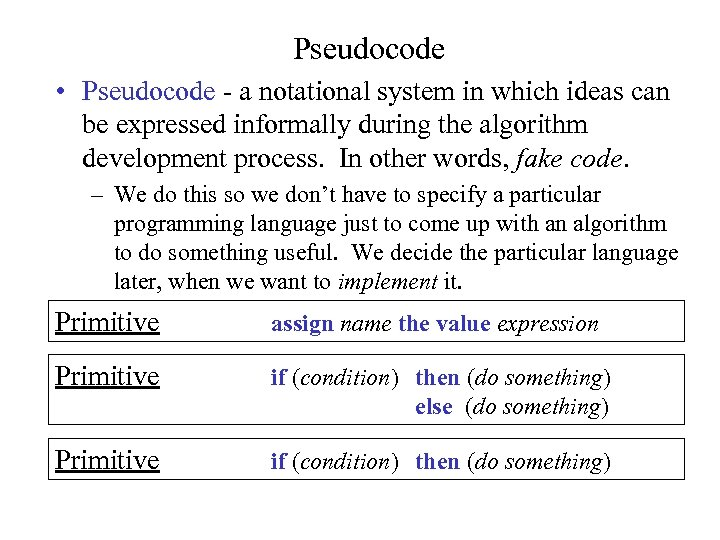Pseudocode • Pseudocode - a notational system in which ideas can be expressed informally during the algorithm development process. In other words, fake code. – We do this so we don’t have to specify a particular programming language just to come up with an algorithm to do something useful. We decide the particular language later, when we want to implement it. Primitive assign name the value expression Primitive if (condition) then (do something) else (do something) Primitive if (condition) then (do something)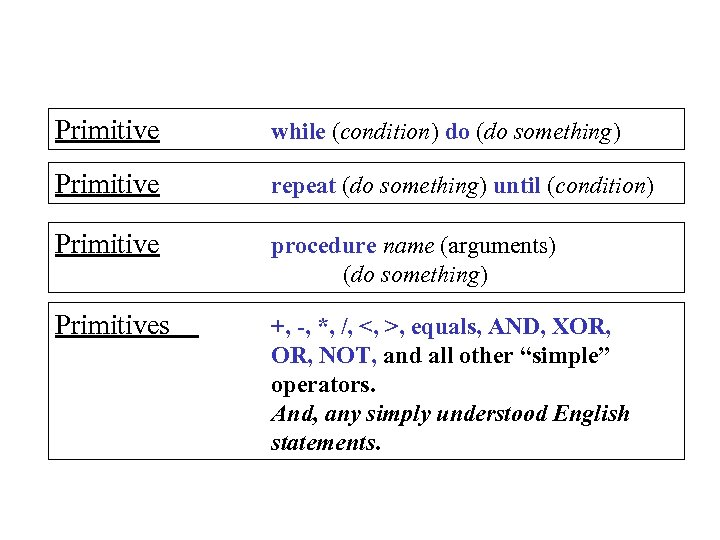Primitive while (condition) do (do something) Primitive repeat (do something) until (condition) Primitive procedure name (arguments) (do something) Primitives +, -, *, /, <, >, equals, AND, XOR, NOT, and all other “simple” operators. And, any simply understood English statements.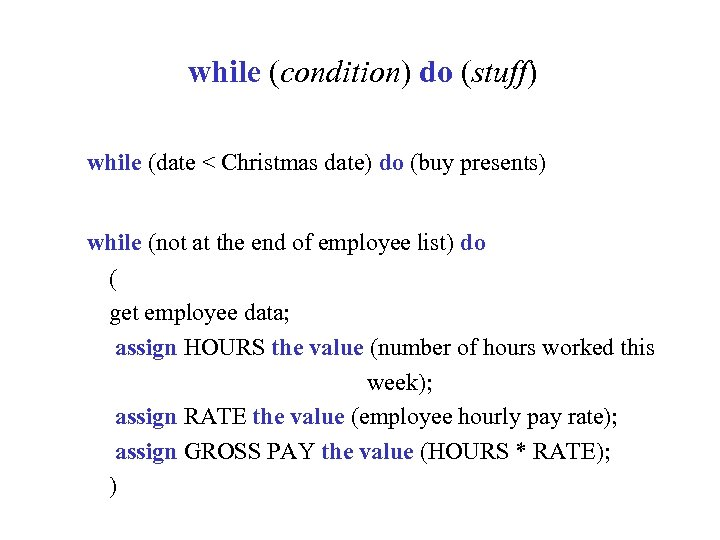while (condition) do (stuff) while (date < Christmas date) do (buy presents) while (not at the end of employee list) do ( get employee data; assign HOURS the value (number of hours worked this week); assign RATE the value (employee hourly pay rate); assign GROSS PAY the value (HOURS * RATE); )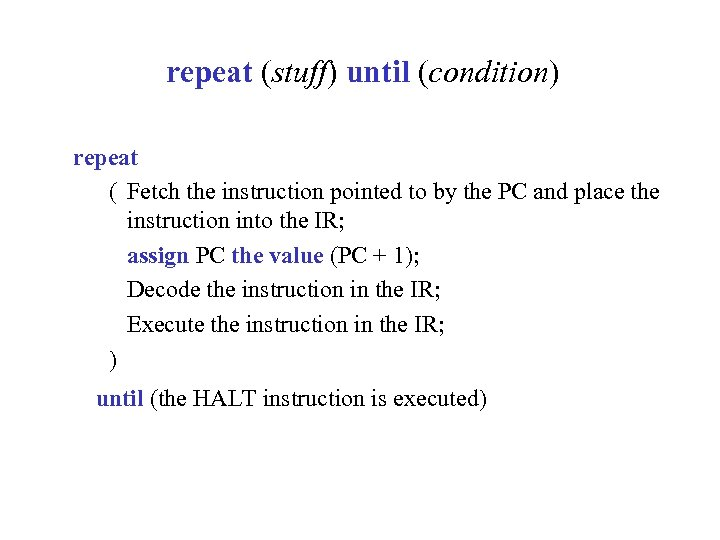repeat (stuff) until (condition) repeat ( Fetch the instruction pointed to by the PC and place the instruction into the IR; assign PC the value (PC + 1); Decode the instruction in the IR; Execute the instruction in the IR; ) until (the HALT instruction is executed)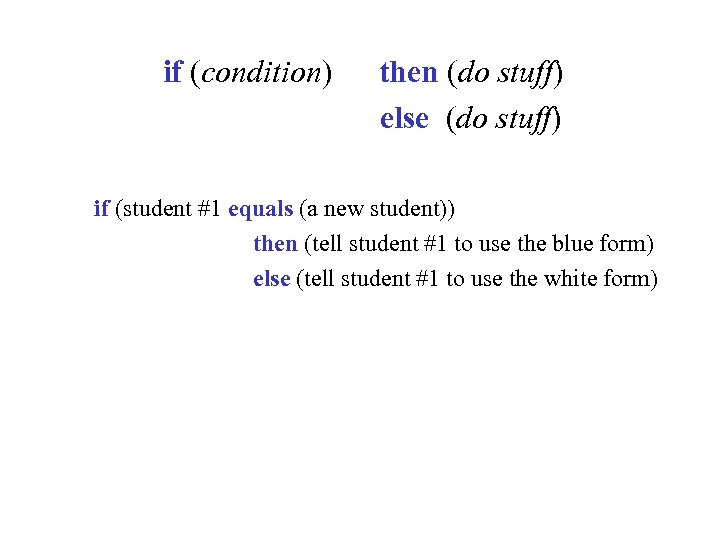if (condition) then (do stuff) else (do stuff) if (student #1 equals (a new student)) then (tell student #1 to use the blue form) else (tell student #1 to use the white form)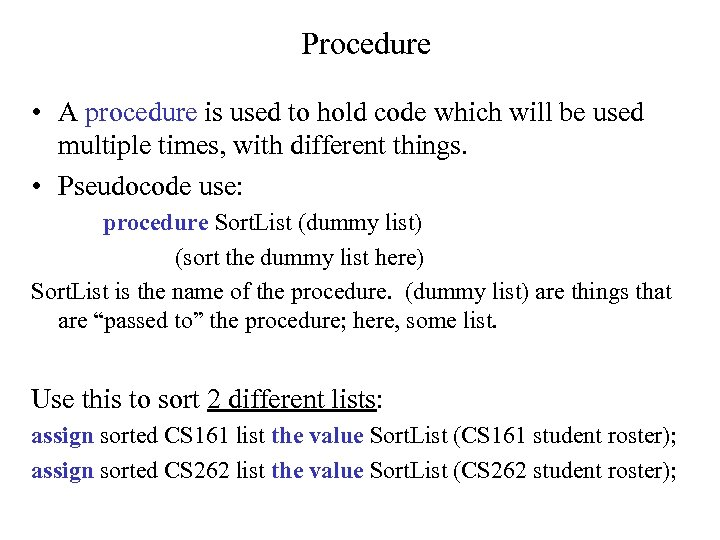Procedure • A procedure is used to hold code which will be used multiple times, with different things. • Pseudocode use: procedure Sort. List (dummy list) (sort the dummy list here) Sort. List is the name of the procedure. (dummy list) are things that are “passed to” the procedure; here, some list. Use this to sort 2 different lists: assign sorted CS 161 list the value Sort. List (CS 161 student roster); assign sorted CS 262 list the value Sort. List (CS 262 student roster);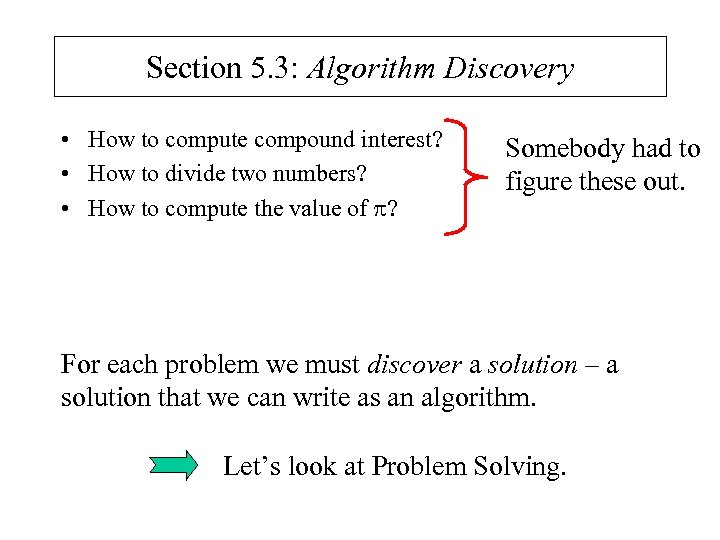Section 5. 3: Algorithm Discovery • How to compute compound interest? • How to divide two numbers? • How to compute the value of ? Somebody had to figure these out. For each problem we must discover a solution – a solution that we can write as an algorithm. Let’s look at Problem Solving.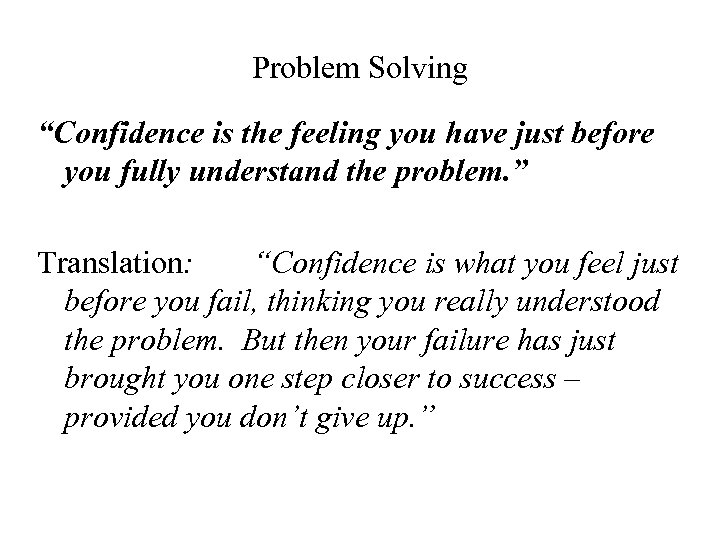Problem Solving “Confidence is the feeling you have just before you fully understand the problem. ” Translation: “Confidence is what you feel just before you fail, thinking you really understood the problem. But then your failure has just brought you one step closer to success – provided you don’t give up. ”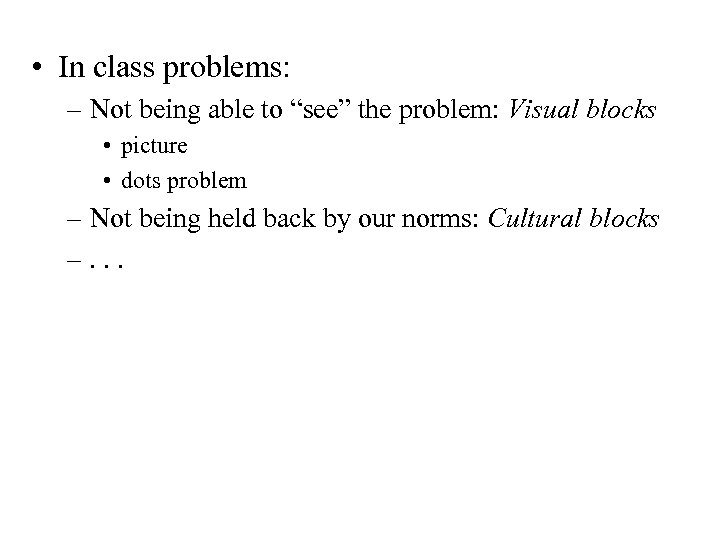• In class problems: – Not being able to “see” the problem: Visual blocks • picture • dots problem – Not being held back by our norms: Cultural blocks –. . .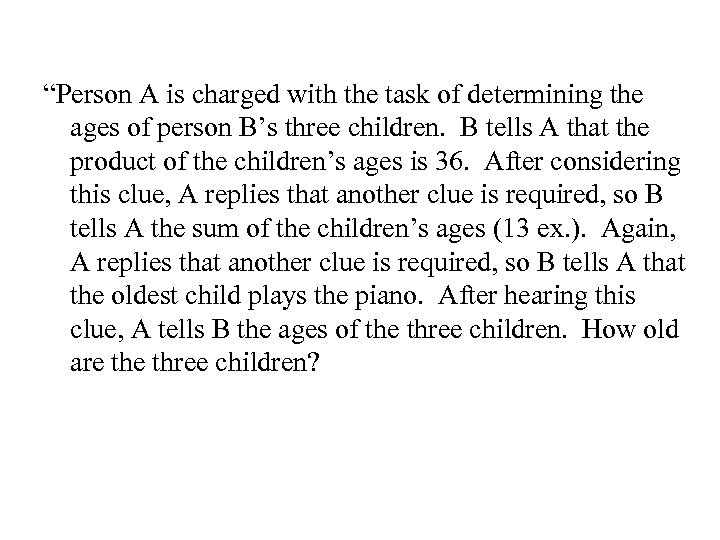“Person A is charged with the task of determining the ages of person B’s three children. B tells A that the product of the children’s ages is 36. After considering this clue, A replies that another clue is required, so B tells A the sum of the children’s ages (13 ex. ). Again, A replies that another clue is required, so B tells A that the oldest child plays the piano. After hearing this clue, A tells B the ages of the three children. How old are three children?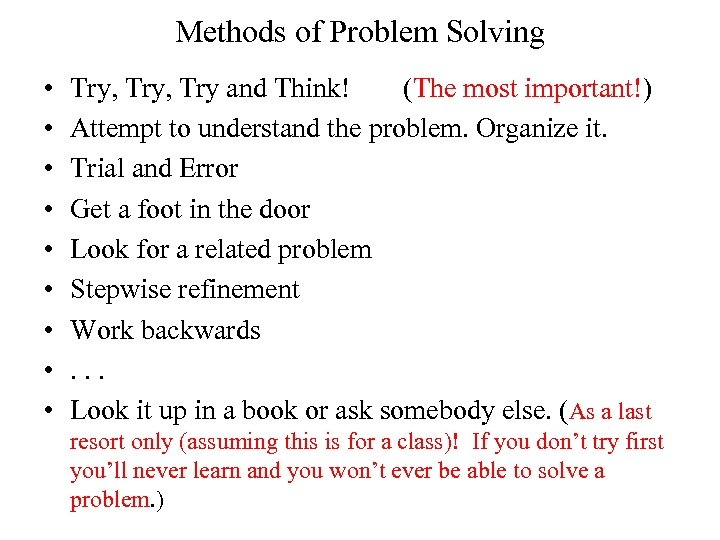Methods of Problem Solving • • • Try, Try and Think! (The most important!) Attempt to understand the problem. Organize it. Trial and Error Get a foot in the door Look for a related problem Stepwise refinement Work backwards. . . Look it up in a book or ask somebody else. (As a last resort only (assuming this is for a class)! If you don’t try first you’ll never learn and you won’t ever be able to solve a problem. )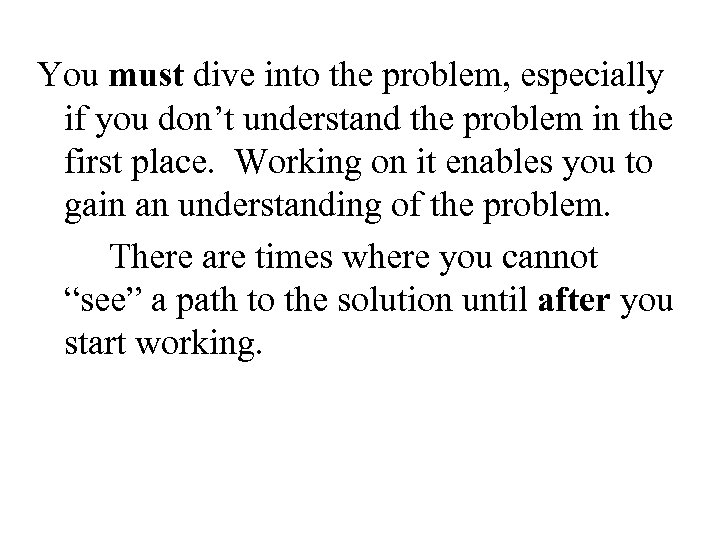You must dive into the problem, especially if you don’t understand the problem in the first place. Working on it enables you to gain an understanding of the problem. There are times where you cannot “see” a path to the solution until after you start working.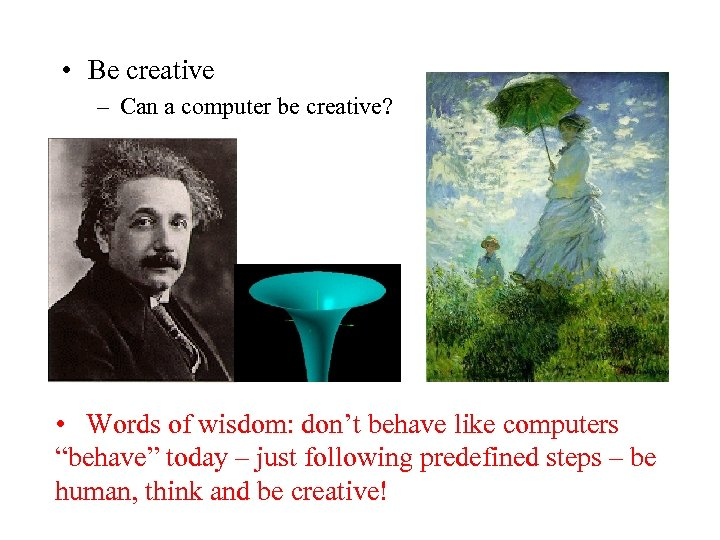• Be creative – Can a computer be creative? • Words of wisdom: don’t behave like computers “behave” today – just following predefined steps – be human, think and be creative!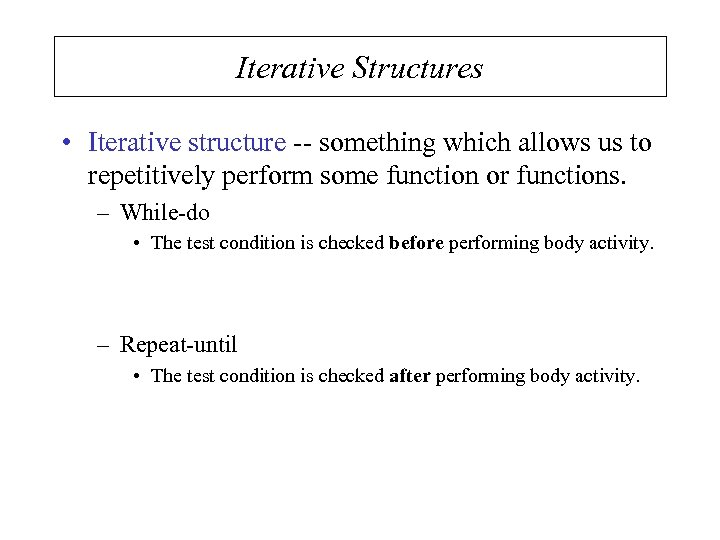Iterative Structures • Iterative structure -- something which allows us to repetitively perform some function or functions. – While-do • The test condition is checked before performing body activity. – Repeat-until • The test condition is checked after performing body activity.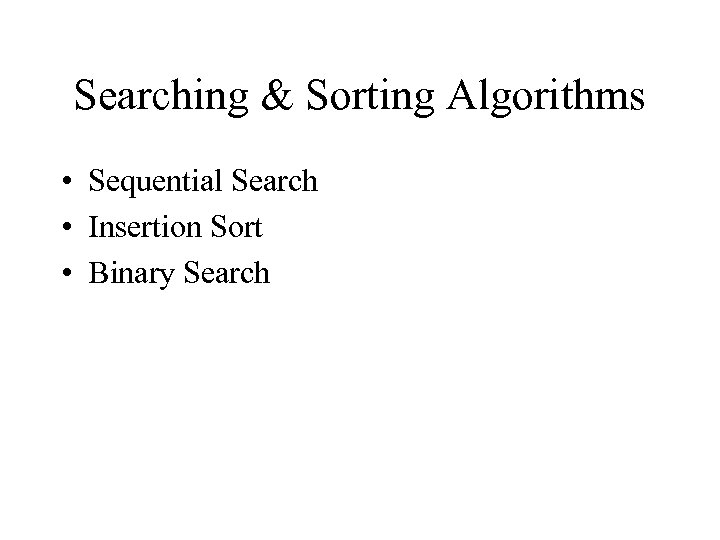Searching & Sorting Algorithms • Sequential Search • Insertion Sort • Binary Search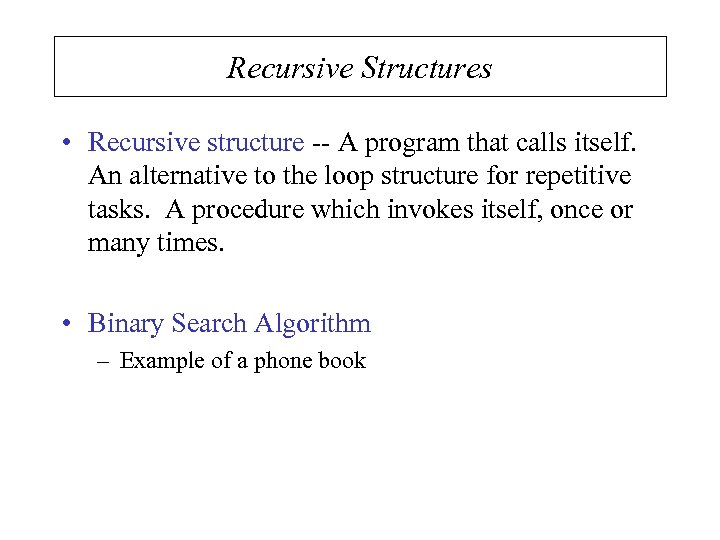Recursive Structures • Recursive structure -- A program that calls itself. An alternative to the loop structure for repetitive tasks. A procedure which invokes itself, once or many times. • Binary Search Algorithm – Example of a phone book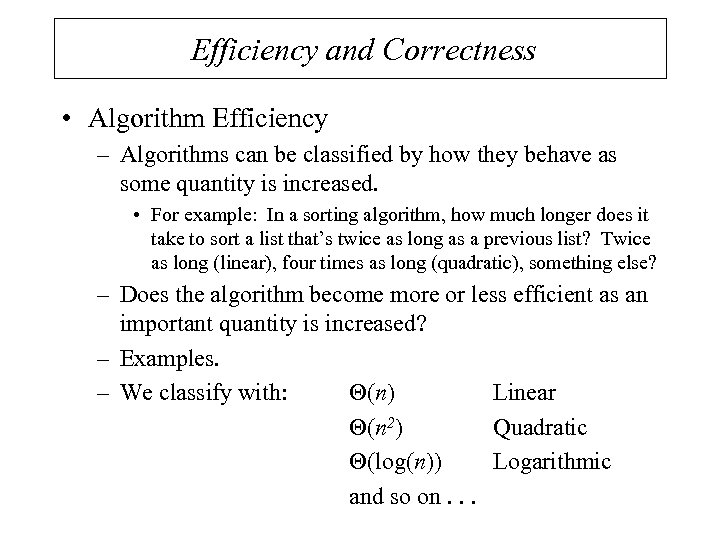Efficiency and Correctness • Algorithm Efficiency – Algorithms can be classified by how they behave as some quantity is increased. • For example: In a sorting algorithm, how much longer does it take to sort a list that’s twice as long as a previous list? Twice as long (linear), four times as long (quadratic), something else? – Does the algorithm become more or less efficient as an important quantity is increased? – Examples. – We classify with: (n) Linear (n 2) Quadratic (log(n)) Logarithmic and so on. . .